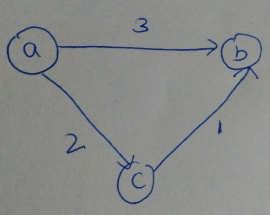# GATE | GATE-CS-2017 (Set 1) | Question 37

• Last Updated : 28 Jun, 2021

Let G = (V, E) be any connected undirected edge-weighted graph. The weights of the edges in E are positive any distinct. Consider the following statements:

```I. Minimum Spanning Tree of G is always unique.
II. Shortest path between any two vertices of G is always unique.
```

Which of the above statements is/are necessarily true?
(A) I only
(B) II only
(C) both I and II
(D) neither I and II

Explanation: I. Minimum Spanning Tree of G is always unique – MST will wlways be distinct if the edges are unique so Correct
II. Shortest path between any two vertices of G is always unique – Shortest path between any two vertices can be same so incorrect

Therefore, option A is correct

Alternate solution:
We know that minimum spanning tree of a graph is always unique if all the weight are distinct, so statement 1 is correct.
Now statement 2 , this might not be true in all cases. Consider the graph.There are two shortest paths from a to b (one is direct and other via node c) So statement 2 is false
Hence option a is correct.

Quiz of this Question

My Personal Notes arrow_drop_up